## Wednesday, November 2, 2016

### Modified version of Grafen's branch lengths

Last night - based on an R-sig-phylo request I wrote some code to compute a modified version of Grafen's edge lengths, in which the depth of each node is defined by the number of branching events above it - not the number of leaves.

However, this is not what I think the commenter wanted. Indeed, I believe he was looking for a transformation in which the height above the root of all nodes with a maximum path length to any tip of x was standardized for a given value of x.

Here is an example of this. First, our functions:

``````node.paths<-function(tree,node){
d<-Descendants(tree,node,"children")
paths<-as.list(d)
while(any(d>Ntip(tree))){
jj<-1
new.paths<-list()
for(i in 1:length(paths)){
if(paths[[i]][length(paths[[i]])]<=Ntip(tree)){
new.paths[[jj]]<-paths[[i]]
jj<-jj+1
} else {
ch<-Descendants(tree,paths[[i]][length(paths[[i]])],
"children")
for(j in 1:length(ch)){
new.paths[[jj]]<-c(paths[[i]],ch[j])
jj<-jj+1
}
}
}
paths<-new.paths
d<-sapply(paths,function(x) x[length(x)])
}
paths
}
modified.Grafen<-function(tree,power=2){
max.np<-function(tree,node){
np<-node.paths(tree,node)
if(length(np)>0) max(sapply(np,length)) else 0
}
nn<-1:(Ntip(tree)+tree\$Nnode)
h<-sapply(nn,max.np,tree=tree)+1
h<-(h/max(h))^power
edge.length<-vector()
for(i in 1:nrow(tree\$edge))
edge.length[i]<-diff(h[tree\$edge[i,2:1]])
tree\$edge.length<-edge.length
tree
}
``````

Now our trees:

``````library(phytools)
library(phangorn)
## standard Grafen tree 1
plotTree(t1,lwd=1)
``````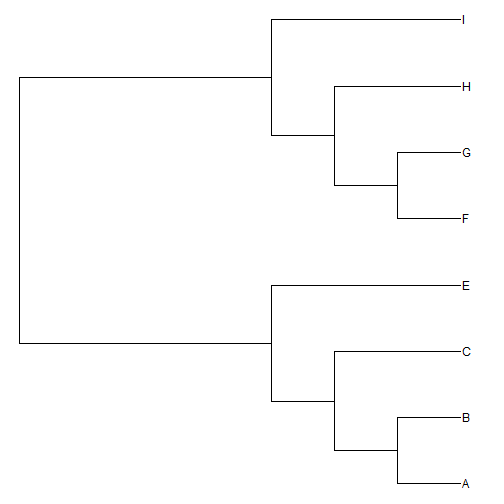``````## modified Grafen
plotTree(modified.Grafen(t1),lwd=1)
``````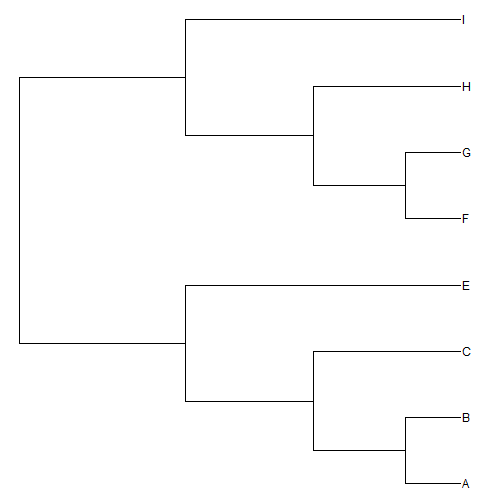``````## standard Grafen tree 2
plotTree(t2,lwd=1)
``````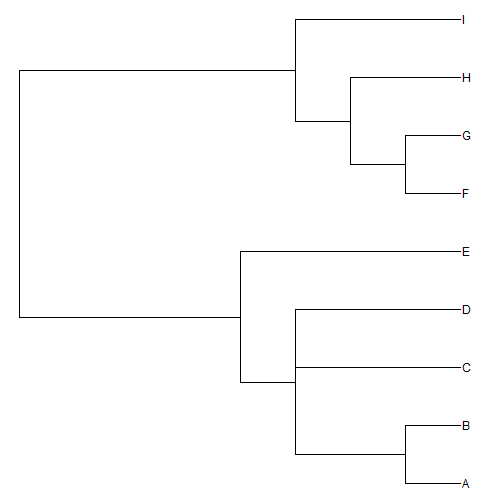``````plotTree(modified.Grafen(t2),lwd=1)
nodelabels()
``````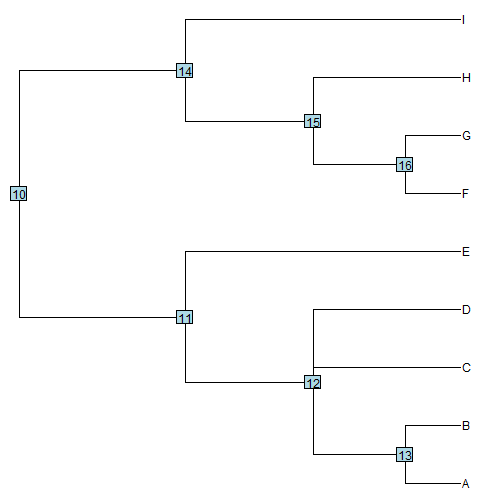Note that nodes #11 & #14 have the same height because they have the same maximum path length to the tips.

``````## standard Grafen tree 3
plotTree(t3,lwd=1)
``````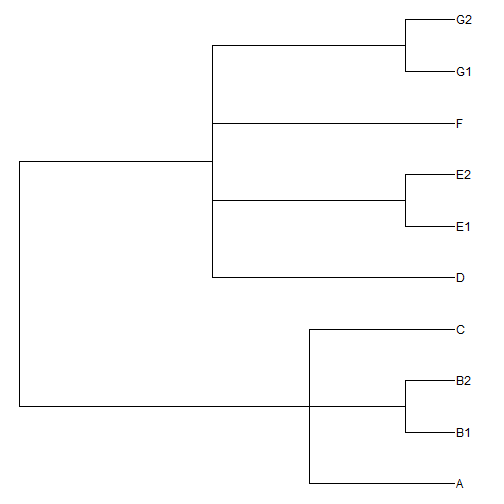``````plotTree(modified.Grafen(t3,power=3),lwd=1)
``````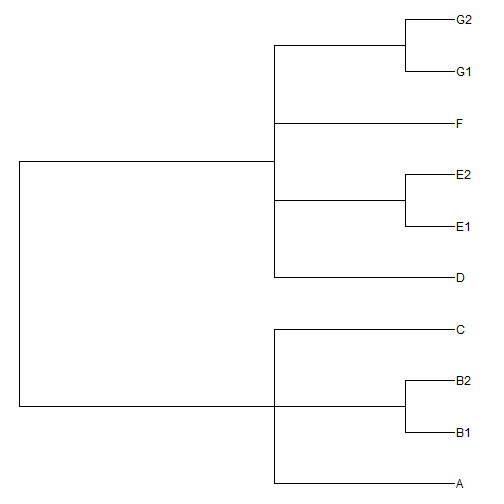Changing `power` just changes how the tree looks - not the fact that nodes a common maximum number of edges away from the present are at a common height.

Finally, here I show the plot with node labels giving the maximum depth (in nodes) of each internal node of the tree. We can see that with `modified.Grafen` nodes with the same index have the same height.

``````tree<-rtree(n=40)
tree\$edge.length[sample(1:nrow(tree\$edge),40)]<-0
tree<-di2multi(tree)
tree\$edge.length<-NULL
max.np<-sapply(1:tree\$Nnode+Ntip(tree),function(x,tree)
max(sapply(node.paths(tree,x),length)),tree=tree)
plotTree(tree,lwd=1,fsize=0.7)
nodelabels(max.np,node=1:tree\$Nnode+Ntip(tree),cex=0.7)
``````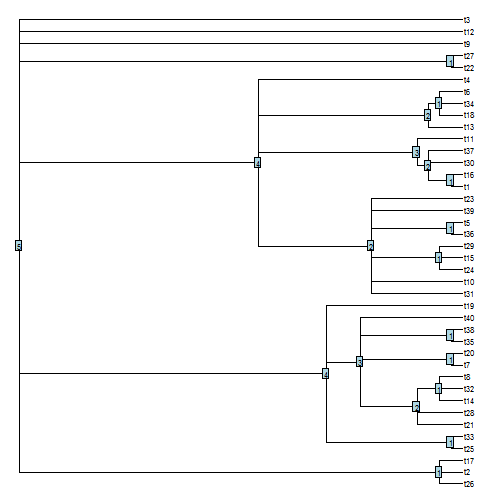``````plotTree(modified.Grafen(tree,power=3),lwd=1,fsize=0.7)
nodelabels(max.np,node=1:tree\$Nnode+Ntip(tree),cex=0.7)
``````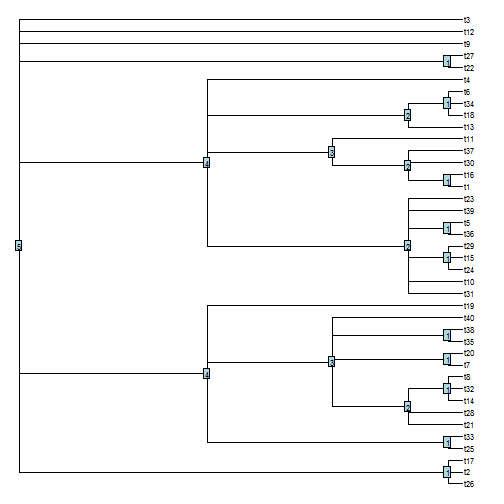That's all.

#### 1 comment:

1.